# High School Math : Rectangles

## Example Questions

1 2 3 5 Next →

### Example Question #6 : How To Find The Area Of A Rectangle

The front façade of a building is 100 feet tall and 40 feet wide.  There are eight floors in the building, and each floor has four glass windows that are 8 feet wide and 6 feet tall along the front façade.  What is the total area of the glass in the façade?

2464 ft2

768 ft2

1536 ft2

192 ft2

1536 ft2

1536 ft2

Explanation:

Glass Area per Window = 8 ft x 6 ft = 48 ft2

Total Number of Windows = Windows per Floor * Number of Floors = 4 * 8 = 32 windows

Total Area of Glass = Area per Window * Total Number of Windows = 48 * 32 = 1536 ft2

### Example Question #1 : How To Find The Area Of A Rectangle

Mark is making a plan to build a rectangular garden.  He has 160 feet of fence to form the outside border of the garden.  He wants the dimensions to look like the plan outlined below: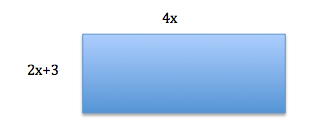What is the area of the garden, rounded to the nearest square foot?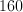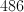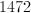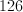Explanation:

Perimeter:  Sum of the sides:

4x + 4x + 2x+8 +2x+8 = 160

12x + 6 = 160

12x = 154

x =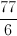Therefore, the short side of the rectangle is going to be: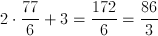And the long side is going to be: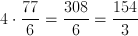The area of the rectangle is going to be as follows:

Area = lw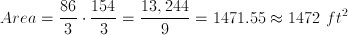### Example Question #191 : Geometry

Two circles of a radius of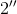each sit inside a square with a side length of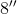.  If the circles do not overlap, what is the area outside of the circles, but within the square?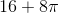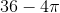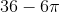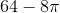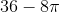Explanation:

The area of a square =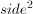The area of a circle is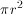Area  = Area of Square2(Area of Circle) =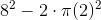### Example Question #198 : Geometry

If the area Rectangle A islarger than Rectangle B and the sides of Rectangle A areand, what is the area of Rectangle B?Explanation: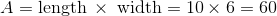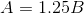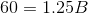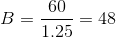### Example Question #231 : Plane Geometry

Erin is getting ready to plant her tulip garden. She wants to plant two tulips per square foot of garden. If her rectangular garden is enclosed by 24 feet of fencing, and the length of the fence is twice as long as its width, how many tulips will Erin plant?

48

32

24

64

16

64

Explanation:

We know that the following represents the formula for the perimeter of a rectangle:In this particular case, we are told that the length of the fence is twice as long as the width. We can write this as the following expression:Use this information to substitute in a variable for the length that matches the variable for width in our perimeter equation.We also know that the length is two times the width; therefore, we can write the following:The area of a rectangle is found by using this formula:The area of the garden is 32 square feet. Erin will plant two tulips per square foot; thus, she will plant 64 tulips.

1 2 3 5 Next →